### American Idiot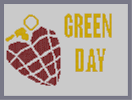Hover over the thumbnail for a full-size version.

Author kamikaze3000 author:kamikaze3000 n-art rated 2006-02-26 4 by 37 people. \$American Idiot#kamikaze3000#none#00000000000000000000000000000000000000000000000000000000000000000000000000000000000000000000000000000000000000000000000000000000000000000000000000000000000000000000000000000000000000000000000000000000000000000000000000000000000000000000000000000000000000000000000000000000000000000000000000000000000000000000000000000000000000000000000000000000000000000000000000000000000000000000000000000000000000000000000000000000000000000000000000000000000000000000000000000000000000000000000000000000000000000000000000000000000000000000000000000000000000000000000000000000000000000000000000000000000000000000000000000000000000000000000000000000000000000000000000000000000000000000000000000000000000000000000000000000000000000|12^42,306!12^48,306!12^42,312!12^48,312!12^54,312!12^42,318!12^48,318!12^54,318!12^60,318!12^66,318!12^42,324!12^48,324!12^54,324!12^60,324!12^66,324!12^72,324!12^84,330!12^78,330!12^72,330!12^66,330!12^60,330!12^48,330!12^54,330!12^42,330!12^90,336!12^84,336!12^78,336!12^72,336!12^66,336!12^60,336!12^48,336!12^54,336!12^42,336!12^84,342!12^78,342!12^72,342!12^66,342!12^60,342!12^54,342!12^48,342!12^48,342!12^42,342!12^42,348!12^48,348!12^60,348!12^54,348!12^72,348!12^66,348!12^78,348!12^72,354!12^66,354!12^66,354!12^60,354!12^60,354!12^54,354!12^48,354!12^42,354!12^66,360!12^60,360!12^54,360!12^48,360!12^54,366!12^60,366!12^54,372!12^60,372!12^66,384!12^72,378!12^72,384!12^72,390!12^78,378!12^78,384!12^78,372!12^78,390!12^78,396!12^84,402!12^84,396!12^84,390!12^84,384!12^84,378!12^84,372!12^84,366!12^90,408!12^90,402!12^90,396!12^90,390!12^90,384!12^90,384!12^90,378!12^90,372!12^90,366!12^90,360!12^96,354!12^96,360!12^96,366!12^96,372!12^96,384!12^96,378!12^96,390!12^96,396!12^96,402!12^96,408!12^96,414!12^102,414!12^102,408!12^102,402!12^102,396!12^102,390!12^102,384!12^102,378!12^102,372!12^102,366!12^102,360!12^102,354!12^102,348!12^108,408!12^108,402!12^108,396!12^108,390!12^108,384!12^108,378!12^108,372!12^108,366!12^108,360!12^108,354!12^114,402!12^114,396!12^114,390!12^114,384!12^114,372!12^114,378!12^114,366!12^114,360!12^120,372!12^120,378!12^120,378!12^120,384!12^120,390!12^120,390!12^120,396!12^120,402!12^126,378!12^126,384!12^126,390!12^126,396!12^132,396!12^132,390!12^132,384!12^138,390!12^138,396!12^114,420!12^114,426!12^120,420!12^120,426!12^120,432!12^126,420!12^126,426!12^126,432!12^126,438!12^132,420!12^132,426!12^132,438!12^132,432!12^132,444!12^138,414!12^138,420!12^138,426!12^138,432!12^138,438!12^138,444!12^144,450!12^144,444!12^144,438!12^144,432!12^144,426!12^144,420!12^144,414!12^150,450!12^150,444!12^156,450!12^156,444!12^156,414!12^150,414!12^150,420!12^150,426!12^150,432!12^150,438!12^156,438!12^156,432!12^156,426!12^156,420!12^162,444!12^162,438!12^162,438!12^162,432!12^162,426!12^162,420!12^168,444!12^168,438!12^168,432!12^168,426!12^174,432!12^174,438!12^174,444!12^168,462!12^174,456!12^174,462!12^174,468!12^180,456!12^180,462!12^180,468!12^186,456!12^186,462!12^186,468!12^186,474!12^192,456!12^192,462!12^192,468!12^192,474!12^198,462!12^198,468!12^198,474!12^198,480!12^204,462!12^204,468!12^204,474!12^204,480!12^210,468!12^210,474!12^210,480!12^210,486!12^216,474!12^216,480!12^216,486!12^216,492!12^222,474!12^222,480!12^222,486!12^222,492!12^228,480!12^228,486!12^228,492!12^234,486!12^234,492!12^240,486!12^240,492!12^246,504!12^252,504!12^258,504!12^258,498!12^264,498!12^264,504!12^270,504!12^276,504!12^282,504!12^90,318!12^84,312!12^78,306!12^72,300!12^66,300!12^60,294!12^54,294!12^54,288!12^54,282!12^54,276!12^60,270!12^60,276!12^60,276!12^60,282!12^60,288!12^66,264!12^66,270!12^66,276!12^66,282!12^66,288!12^66,294!12^72,264!12^72,270!12^72,276!12^72,282!12^72,288!12^72,294!12^78,300!12^78,288!12^78,288!12^78,294!12^78,282!12^78,276!12^78,270!12^78,264!12^84,258!12^84,264!12^84,270!12^84,270!12^84,300!12^84,306!12^84,288!12^84,294!12^84,282!12^84,276!12^90,312!12^90,306!12^90,300!12^90,294!12^90,288!12^90,282!12^90,276!12^90,270!12^90,258!12^90,264!12^96,318!12^96,312!12^96,306!12^96,300!12^96,294!12^96,288!12^96,282!12^96,276!12^96,276!12^96,276!12^96,270!12^96,264!12^96,258!12^102,252!12^102,318!12^108,312!12^108,252!12^114,306!12^114,252!12^120,300!12^120,252!12^126,258!12^126,294!12^132,294!12^132,258!12^138,258!12^138,282!12^138,288!12^144,258!12^144,282!12^144,288!12^150,282!12^150,258!12^156,258!12^156,282!12^162,264!12^162,270!12^162,276!12^168,270!12^168,276!12^174,270!12^102,258!12^108,258!12^114,258!12^120,258!12^108,264!12^102,264!12^114,264!12^120,264!12^126,264!12^132,264!12^138,264!12^150,264!12^156,264!12^150,264!12^144,264!12^162,270!12^102,270!12^108,270!12^120,270!12^126,270!12^144,270!12^120,270!12^114,270!12^126,270!12^144,270!12^138,270!12^132,270!12^150,270!12^150,270!12^162,270!12^156,270!12^156,276!12^150,276!12^138,276!12^138,276!12^144,276!12^126,276!12^120,276!12^114,276!12^108,276!12^108,276!12^132,276!12^108,276!12^102,276!12^102,282!12^108,282!12^114,282!12^120,282!12^126,282!12^132,282!12^132,288!12^120,288!12^120,288!12^114,288!12^108,288!12^102,288!12^102,288!12^126,288!12^120,294!12^102,294!12^102,294!12^114,294!12^114,294!12^108,294!12^102,306!12^108,300!12^102,300!12^114,300!12^108,306!12^102,312!12^108,336!12^114,342!12^114,336!12^114,330!12^120,324!12^120,330!12^120,336!12^120,342!12^120,348!12^126,318!12^126,324!12^126,330!12^126,330!12^126,336!12^126,342!12^126,348!12^126,354!12^132,312!12^132,360!12^138,312!12^138,366!12^144,306!12^144,372!12^150,300!12^150,378!12^150,384!12^156,384!12^156,300!12^162,300!12^162,378!12^168,294!12^168,372!12^174,372!12^174,294!12^180,288!12^180,366!12^186,288!12^186,360!12^192,360!12^192,294!12^192,300!12^198,306!12^198,354!12^204,348!12^204,312!12^210,348!12^210,342!12^210,336!12^210,330!12^210,324!12^204,318!12^216,342!12^216,336!12^216,330!12^222,336!12^222,342!12^168,324!12^156,324!12^156,342!12^162,342!12^162,336!12^162,330!12^156,330!12^150,330!12^144,330!12^144,336!12^156,336!12^156,336!12^156,336!12^150,336!12^174,330!12^168,330!12^168,330!12^162,324!12^162,324!12^180,330!12^180,330!12^174,342!12^174,342!12^168,336!12^180,336!12^180,342!12^180,342!12^186,336!12^186,336!12^180,336!12^174,336!12^174,342!12^168,348!12^162,348!12^162,354!12^168,348!12^174,318!12^168,342!12^132,330!12^132,330!12^138,330!12^138,336!12^132,336!12^132,336!12^132,342!12^138,342!12^150,342!12^150,342!12^144,342!12^132,348!12^138,348!12^144,348!12^150,348!12^156,348!12^168,348!12^168,360!12^156,360!12^156,348!12^156,354!12^150,348!12^150,354!12^144,354!12^138,354!12^132,354!12^138,360!12^144,360!12^150,360!12^162,360!12^168,354!12^174,348!12^180,348!12^138,324!12^138,318!12^132,318!12^132,324!12^144,324!12^144,318!12^156,318!12^150,324!12^150,324!12^150,318!12^150,312!12^144,312!12^150,306!12^162,306!12^162,312!12^156,312!12^162,306!12^162,306!12^162,306!12^156,306!12^162,312!12^162,318!12^174,318!12^174,306!12^174,312!12^180,324!12^186,324!12^192,324!12^192,330!12^192,330!12^204,330!12^186,330!12^198,330!12^198,330!12^144,366!12^150,366!12^156,366!12^150,372!12^156,378!12^156,372!12^162,372!12^162,366!12^168,366!12^174,360!12^174,366!12^174,354!12^180,360!12^180,354!12^186,354!12^186,348!12^186,342!12^192,354!12^192,348!12^192,342!12^192,336!12^198,348!12^198,336!12^198,336!12^198,342!12^204,342!12^204,336!12^168,300!12^168,306!12^168,312!12^168,324!12^174,318!12^168,318!12^174,324!12^174,300!12^180,294!12^180,300!12^180,306!12^180,312!12^180,312!12^180,312!12^180,318!12^186,318!12^186,306!12^186,312!12^186,300!12^186,300!12^186,294!12^192,306!12^192,312!12^192,312!12^192,318!12^198,312!12^198,312!12^198,318!12^198,318!12^204,324!12^198,324!12^132,414!12^168,402!12^168,396!12^174,396!12^174,396!12^174,402!12^174,408!12^174,414!12^180,420!12^180,414!12^180,408!12^180,402!12^180,396!12^186,426!12^186,420!12^186,414!12^186,408!12^186,402!12^186,396!12^186,390!12^192,384!12^192,390!12^192,396!12^192,402!12^192,402!12^192,408!12^192,414!12^192,420!12^192,426!12^192,426!12^192,432!12^198,378!12^198,384!12^198,390!12^198,396!12^198,402!12^198,408!12^198,414!12^198,420!12^198,426!12^198,432!12^204,378!12^204,390!12^204,384!12^204,396!12^204,402!12^204,402!12^204,408!12^204,414!12^204,420!12^204,426!12^204,432!12^210,372!12^210,378!12^210,384!12^210,390!12^210,396!12^210,402!12^210,408!12^210,408!12^210,414!12^210,420!12^210,426!12^216,420!12^216,414!12^216,408!12^216,402!12^216,396!12^216,390!12^216,390!12^216,384!12^216,378!12^216,372!12^222,366!12^222,420!12^222,414!12^222,408!12^222,402!12^222,396!12^222,390!12^222,384!12^222,378!12^222,372!12^228,360!12^234,360!12^228,414!12^234,414!12^228,408!12^228,402!12^228,402!12^228,396!12^228,390!12^228,390!12^228,384!12^228,378!12^228,372!12^228,366!12^234,366!12^234,372!12^234,378!12^234,378!12^234,384!12^234,390!12^234,390!12^234,396!12^234,402!12^234,408!12^138,408!12^144,408!12^150,408!12^240,378!12^240,408!12^240,402!12^240,396!12^240,390!12^240,384!12^246,384!12^246,390!12^246,396!12^246,396!12^246,402!12^252,396!12^252,402!12^210,450!12^216,450!12^216,444!12^222,444!12^222,450!12^222,456!12^228,462!12^228,456!12^228,450!12^228,444!12^228,438!12^234,462!12^234,456!12^234,450!12^234,444!12^234,438!12^240,468!12^240,462!12^240,456!12^240,450!12^240,444!12^240,438!12^240,432!12^246,426!12^246,432!12^246,438!12^246,444!12^246,450!12^246,456!12^246,462!12^246,468!12^252,420!12^252,474!12^252,468!12^252,462!12^252,456!12^252,450!12^252,444!12^252,438!12^252,432!12^252,426!12^258,480!12^258,414!12^264,414!12^264,486!12^270,480!12^270,426!12^264,420!12^276,474!12^276,432!12^276,438!12^282,444!12^282,468!12^282,450!12^288,456!12^288,462!12^282,462!12^282,450!12^282,456!12^258,420!12^258,426!12^258,426!12^264,426!12^258,426!12^258,432!12^258,438!12^258,444!12^258,450!12^258,456!12^258,462!12^258,468!12^258,474!12^264,480!12^264,474!12^264,468!12^264,456!12^264,450!12^264,438!12^264,438!12^264,432!12^264,438!12^264,462!12^264,444!12^270,432!12^270,438!12^270,444!12^276,444!12^270,450!12^276,450!12^264,456!12^276,456!12^270,456!12^276,462!12^270,462!12^270,468!12^276,468!12^270,474!12^282,492!12^288,492!12^294,486!12^294,492!12^294,498!12^300,480!12^300,486!12^300,492!12^300,498!12^306,474!12^306,480!12^306,486!12^306,492!12^306,498!12^312,468!12^312,474!12^312,480!12^312,486!12^312,492!12^312,498!12^312,504!12^318,468!12^318,474!12^318,480!12^318,486!12^318,492!12^318,498!12^318,504!12^324,510!12^324,468!12^324,474!12^324,480!12^324,486!12^324,492!12^324,498!12^324,498!12^324,498!12^324,504!12^330,510!12^330,504!12^330,498!12^330,492!12^330,486!12^330,480!12^330,474!12^330,468!12^330,462!12^336,462!12^336,504!12^336,498!12^336,492!12^336,492!12^336,486!12^336,480!12^336,474!12^336,468!12^348,462!12^348,468!12^348,474!12^348,456!12^348,450!12^354,450!12^348,444!12^354,444!12^354,438!12^276,402!12^282,402!12^282,408!12^288,414!12^288,420!12^288,396!12^288,402!12^288,408!12^294,426!12^294,432!12^294,420!12^294,414!12^294,408!12^294,402!12^294,396!12^300,438!12^300,438!12^300,444!12^300,390!12^300,396!12^300,402!12^300,402!12^300,408!12^300,414!12^300,420!12^300,426!12^300,432!12^306,450!12^306,390!12^306,402!12^306,396!12^306,408!12^306,420!12^306,414!12^306,426!12^306,432!12^306,438!12^306,444!12^312,384!12^312,390!12^312,396!12^312,402!12^312,408!12^312,414!12^312,420!12^312,426!12^312,432!12^312,438!12^312,438!12^312,444!12^312,450!12^318,378!12^324,378!12^318,444!12^324,444!12^318,438!12^318,432!12^318,432!12^318,426!12^318,420!12^318,414!12^318,414!12^318,408!12^318,402!12^318,396!12^318,396!12^318,390!12^318,390!12^318,384!12^324,384!12^324,390!12^324,396!12^324,402!12^324,402!12^324,408!12^324,414!12^324,414!12^324,414!12^324,420!12^324,420!12^318,426!12^318,432!12^324,426!12^324,432!12^324,432!12^324,438!12^330,438!12^330,432!12^330,426!12^330,420!12^330,414!12^348,420!12^348,414!12^354,414!12^360,408!12^354,408!12^348,408!12^342,408!12^342,402!12^342,396!12^342,390!12^342,384!12^342,378!12^360,378!12^348,378!12^354,378!12^360,384!12^354,390!12^360,390!12^360,396!12^360,402!12^354,402!12^348,402!12^348,396!12^354,396!12^354,390!12^348,390!12^348,384!12^348,384!12^354,384!12^336,372!12^336,366!12^342,366!12^348,360!12^354,354!12^360,348!12^360,354!12^360,360!12^360,366!12^360,372!12^354,372!12^348,372!12^348,372!12^342,372!12^354,366!12^354,360!12^348,366!12^354,432!12^360,432!12^282,510!12^288,510!12^294,510!12^360,426!12^276,390!12^270,390!12^270,384!12^264,378!12^258,372!12^258,366!12^252,360!12^252,354!12^252,348!12^246,348!12^258,342!12^258,348!12^258,354!12^258,360!12^264,366!12^264,372!12^264,360!12^264,354!12^264,348!12^264,342!12^270,336!12^270,342!12^270,348!12^270,348!12^270,354!12^270,354!12^270,360!12^270,366!12^270,366!12^270,372!12^270,378!12^276,336!12^276,342!12^276,342!12^276,348!12^276,348!12^276,354!12^276,360!12^276,360!12^276,366!12^276,372!12^276,372!12^276,378!12^276,384!12^282,384!12^282,378!12^282,372!12^282,366!12^282,360!12^282,354!12^282,348!12^282,342!12^282,336!12^282,330!12^288,324!12^288,330!12^288,378!12^288,372!12^288,366!12^288,360!12^288,354!12^288,354!12^288,348!12^288,342!12^288,336!12^294,378!12^294,318!12^294,324!12^300,330!12^294,330!12^294,336!12^294,336!12^294,342!12^294,348!12^294,354!12^294,360!12^294,366!12^294,372!12^300,372!12^300,366!12^300,360!12^300,354!12^300,348!12^300,342!12^300,336!12^300,324!12^300,318!12^306,366!12^306,360!12^306,354!12^306,348!12^306,342!12^306,330!12^306,324!12^306,318!12^306,312!12^306,336!12^312,318!12^312,324!12^312,330!12^312,336!12^312,336!12^312,342!12^312,348!12^312,354!12^312,354!12^312,360!12^312,366!12^318,360!12^318,354!12^318,348!12^318,342!12^318,336!12^318,300!12^324,300!12^324,306!12^324,312!12^324,318!12^330,294!12^330,324!12^330,330!12^330,318!12^330,312!12^330,306!12^330,300!12^336,294!12^336,336!12^336,342!12^336,348!12^336,330!12^336,324!12^336,318!12^336,312!12^336,306!12^336,300!12^342,288!12^342,342!12^342,336!12^342,330!12^342,324!12^342,318!12^342,312!12^342,306!12^342,306!12^342,300!12^342,294!12^348,288!12^348,336!12^348,330!12^348,324!12^348,324!12^348,318!12^348,312!12^348,306!12^348,300!12^348,300!12^348,294!12^354,282!12^354,330!12^354,324!12^354,318!12^354,312!12^354,306!12^354,294!12^354,288!12^354,300!12^360,288!12^360,330!12^360,324!12^360,318!12^360,312!12^360,306!12^360,306!12^360,300!12^360,294!12^204,282!12^204,276!12^210,270!12^210,294!12^210,288!12^210,282!12^210,276!12^216,300!12^216,294!12^216,288!12^216,282!12^216,276!12^216,276!12^216,270!12^216,264!12^222,258!12^222,264!12^222,270!12^222,276!12^222,282!12^222,288!12^222,294!12^222,300!12^222,306!12^228,252!12^228,312!12^228,306!12^228,300!12^228,294!12^228,288!12^228,282!12^228,276!12^228,270!12^228,264!12^228,264!12^228,258!12^234,318!12^234,324!12^234,312!12^240,306!12^234,306!12^234,300!12^234,300!12^234,288!12^234,294!12^234,282!12^234,276!12^234,270!12^234,264!12^234,258!12^234,252!12^234,246!12^240,330!12^240,240!12^240,246!12^240,252!12^240,264!12^240,258!12^240,270!12^240,282!12^240,276!12^240,294!12^240,288!12^240,306!12^240,300!12^240,312!12^240,318!12^240,324!12^246,240!12^246,246!12^246,252!12^246,258!12^246,264!12^246,270!12^246,282!12^246,276!12^246,330!12^246,324!12^246,318!12^246,312!12^246,306!12^246,300!12^246,294!12^246,288!12^252,324!12^252,234!12^252,246!12^252,240!12^252,252!12^252,258!12^252,264!12^252,270!12^252,276!12^252,282!12^252,288!12^252,294!12^252,306!12^252,306!12^252,300!12^252,312!12^252,318!12^258,234!12^258,318!12^258,312!12^258,300!12^258,306!12^258,288!12^258,294!12^258,276!12^258,282!12^258,264!12^258,270!12^258,258!12^258,252!12^258,246!12^258,240!12^264,228!12^264,234!12^264,240!12^264,240!12^264,246!12^264,246!12^264,252!12^264,258!12^264,264!12^264,270!12^264,276!12^264,288!12^264,288!12^264,288!12^264,282!12^264,294!12^264,294!12^264,300!12^264,300!12^264,306!12^264,306!12^264,312!12^270,306!12^270,300!12^270,294!12^270,288!12^270,228!12^270,234!12^270,234!12^270,240!12^270,246!12^270,258!12^270,252!12^270,264!12^270,270!12^270,282!12^270,276!12^276,222!12^276,228!12^276,234!12^276,240!12^276,246!12^276,252!12^276,258!12^276,264!12^276,270!12^276,276!12^276,282!12^276,294!12^276,288!12^276,300!12^276,306!12^282,300!12^282,294!12^282,288!12^282,282!12^282,276!12^282,270!12^282,264!12^282,258!12^282,252!12^282,246!12^282,240!12^282,234!12^282,228!12^282,222!12^288,300!12^288,234!12^288,240!12^288,246!12^288,252!12^288,264!12^288,258!12^288,270!12^288,276!12^288,282!12^288,288!12^288,288!12^288,294!12^294,294!12^294,258!12^294,264!12^294,270!12^294,276!12^294,282!12^294,288!12^300,294!12^300,288!12^300,282!12^300,276!12^300,222!12^300,228!12^306,234!12^306,240!12^306,246!12^306,252!12^306,228!12^306,222!12^312,258!12^312,264!12^312,270!12^312,276!12^312,252!12^312,246!12^312,240!12^312,234!12^312,228!12^312,222!12^318,228!12^318,282!12^318,276!12^318,270!12^318,264!12^318,258!12^318,252!12^318,252!12^318,246!12^318,240!12^318,234!12^324,276!12^324,270!12^324,264!12^324,258!12^324,252!12^324,246!12^324,240!12^324,234!12^324,228!12^330,276!12^330,270!12^330,264!12^330,258!12^330,246!12^330,252!12^330,240!12^330,234!12^330,228!12^336,228!12^336,228!12^336,234!12^336,240!12^336,246!12^336,252!12^336,258!12^336,258!12^336,264!12^336,270!12^342,228!12^342,240!12^342,234!12^342,246!12^342,246!12^342,252!12^342,258!12^342,264!12^348,240!12^348,246!12^348,252!12^348,252!12^348,258!12^348,264!12^354,258!12^300,198!12^300,192!12^300,186!12^300,180!12^306,186!12^306,192!12^306,198!12^306,198!12^306,204!12^312,204!12^312,198!12^312,192!12^312,192!12^312,186!12^312,186!12^318,186!12^318,192!12^318,198!12^318,198!12^318,204!12^324,192!12^324,192!12^324,198!12^324,198!12^324,204!12^330,204!12^330,198!12^192,264!12^192,258!12^192,252!12^186,246!12^186,240!12^192,234!12^192,228!12^192,222!12^192,240!12^192,246!12^198,216!12^198,210!12^198,204!12^198,198!12^198,258!12^198,252!12^198,246!12^198,240!12^198,240!12^198,234!12^198,228!12^198,222!12^204,252!12^204,192!12^204,198!12^204,204!12^204,210!12^204,216!12^204,222!12^204,222!12^204,228!12^204,234!12^204,234!12^204,240!12^204,246!12^210,186!12^210,246!12^210,234!12^210,240!12^210,228!12^210,222!12^210,216!12^210,210!12^210,204!12^210,198!12^210,192!12^216,186!12^216,240!12^216,234!12^216,228!12^216,222!12^216,216!12^216,216!12^216,210!12^216,204!12^216,198!12^216,192!12^222,180!12^222,234!12^222,228!12^222,222!12^222,216!12^222,210!12^222,210!12^222,204!12^222,204!12^222,198!12^222,198!12^222,192!12^222,186!12^228,180!12^228,228!12^228,222!12^228,216!12^228,210!12^228,204!12^228,198!12^228,192!12^228,186!12^234,174!12^234,180!12^234,186!12^234,192!12^234,192!12^234,198!12^234,198!12^234,222!12^234,216!12^234,210!12^234,204!12^240,168!12^240,174!12^240,180!12^240,180!12^240,186!12^240,192!12^240,198!12^240,204!12^240,210!12^240,216!12^246,216!12^246,210!12^246,204!12^246,198!12^246,192!12^246,186!12^246,180!12^246,174!12^246,168!12^246,162!12^252,210!12^252,204!12^252,198!12^252,192!12^252,186!12^252,180!12^252,174!12^252,168!12^252,162!12^258,168!12^258,174!12^258,180!12^258,180!12^258,180!12^258,186!12^258,192!12^258,192!12^258,198!12^258,204!12^258,210!12^264,168!12^264,174!12^264,180!12^264,186!12^264,192!12^264,192!12^264,198!12^264,204!12^264,204!12^264,210!12^270,204!12^270,198!12^270,192!12^270,186!12^270,180!12^270,174!12^276,174!12^276,180!12^276,186!12^276,186!12^276,192!12^276,198!12^276,204!12^282,186!12^282,192!12^282,198!12^282,204!0^162,240!0^156,240!0^150,234!0^138,234!0^144,234!0^168,234!0^156,234!0^162,234!0^132,228!0^138,228!0^138,228!0^144,228!0^156,228!0^150,228!0^162,228!0^168,228!0^132,222!0^132,216!0^168,216!0^168,222!0^162,222!0^162,216!0^156,216!0^150,216!0^144,216!0^138,216!0^138,222!0^144,222!0^150,222!0^156,222!0^108,216!0^102,216!0^102,222!0^102,210!0^108,210!0^114,210!0^120,210!0^126,210!0^132,210!0^138,210!0^144,210!0^150,210!0^156,210!0^162,210!0^168,210!0^162,204!0^96,204!0^102,204!0^108,204!0^114,204!0^120,204!0^126,204!0^132,204!0^138,204!0^144,204!0^144,204!0^150,204!0^156,204!0^96,198!0^102,198!0^108,198!0^114,198!0^126,198!0^120,198!0^132,198!0^138,198!0^144,198!0^150,198!0^150,192!0^144,192!0^138,192!0^132,192!0^126,192!0^120,192!0^114,192!0^108,192!0^102,192!0^96,192!0^156,186!0^150,186!0^144,186!0^144,186!0^138,186!0^132,186!0^132,186!0^126,186!0^120,186!0^120,186!0^114,186!0^114,186!0^108,186!0^108,186!0^102,186!0^96,186!0^150,180!0^102,180!0^108,180!0^114,180!0^126,180!0^120,180!0^132,180!0^138,180!0^144,180!0^144,174!0^114,174!0^120,174!0^126,174!0^132,174!0^144,174!0^138,174!0^126,168!0^138,168!0^132,168!0^84,216!0^84,210!0^78,216!0^72,216!0^72,222!0^66,222!0^66,228!0^60,222!0^60,228!0^60,234!0^60,234!0^54,228!0^54,234!0^48,234!0^48,228!0^48,240!0^42,234!0^150,150!0^150,144!0^150,138!0^150,132!0^150,126!0^150,126!0^150,120!0^150,114!0^144,120!0^144,120!0^144,126!0^144,132!0^156,150!0^156,156!0^162,162!0^168,162!0^168,168!0^174,168!0^174,174!0^180,174!0^186,174!0^186,180!0^192,180!0^192,174!0^198,168!0^204,168!0^204,168!0^204,162!0^210,162!0^210,156!0^216,156!0^216,150!0^156,114!0^156,108!0^162,108!0^162,102!0^168,102!0^168,96!0^174,96!0^180,96!0^186,96!0^186,90!0^192,90!0^192,96!0^198,96!0^204,96!0^204,102!0^210,102!0^210,108!0^216,108!0^216,114!0^222,114!0^222,120!0^222,126!0^222,132!0^222,138!0^222,144!0^222,150!12^348,480!12^354,456!12^354,462!12^360,438!12^348,486!12^354,468!12^360,444!12^252,498!12^276,510!12^300,510!0^408,84!0^414,84!0^402,90!0^408,90!0^414,90!0^420,90!0^426,96!0^414,96!0^420,96!0^408,96!0^402,96!0^396,96!0^390,102!0^426,102!0^420,102!0^414,102!0^408,102!0^402,102!0^396,102!0^402,108!0^396,108!0^390,108!0^420,108!0^426,108!0^420,114!0^426,114!0^420,120!0^426,120!0^432,120!0^432,120!0^432,126!0^432,132!0^426,132!0^420,132!0^420,126!0^426,126!0^402,114!0^390,120!0^402,126!0^390,132!0^402,138!0^390,144!0^402,150!0^390,156!0^402,162!0^390,168!0^402,174!0^390,180!0^402,186!0^390,192!0^402,198!0^390,204!0^396,204!0^402,204!0^390,198!0^390,186!0^390,180!0^390,174!0^390,162!0^390,150!0^390,138!0^390,126!0^390,114!0^402,120!0^396,114!0^396,120!0^396,126!0^396,132!0^396,138!0^396,144!0^396,150!0^396,156!0^396,162!0^396,168!0^396,174!0^396,186!0^396,186!0^396,192!0^396,192!0^396,180!0^396,198!0^402,192!0^402,186!0^402,180!0^402,168!0^402,162!0^402,156!0^402,144!0^402,132!0^396,210!0^396,216!0^402,216!0^402,210!0^408,210!0^408,216!0^408,222!0^414,222!0^414,222!0^414,216!0^414,210!0^420,210!0^420,216!0^426,216!0^432,204!0^426,210!0^432,210!0^420,204!0^426,204!0^420,198!0^420,198!0^420,192!0^420,186!0^420,180!0^420,174!0^426,174!0^432,174!0^432,180!0^432,180!0^432,186!0^432,192!0^426,198!0^432,198!0^426,192!0^426,186!0^426,180!0^414,168!0^414,162!0^414,156!0^420,156!0^420,162!0^420,168!0^426,168!0^426,162!0^426,156!0^426,156!0^432,156!0^432,162!0^432,168!0^450,222!0^456,222!0^462,222!0^450,210!0^456,210!0^462,210!0^450,198!0^456,198!0^462,198!0^450,186!0^456,186!0^462,186!0^450,174!0^456,174!0^462,174!0^450,162!0^456,162!0^462,162!0^474,162!0^480,162!0^486,162!0^480,168!0^486,168!0^480,174!0^486,174!0^492,174!0^492,180!0^492,186!0^492,192!0^492,198!0^492,204!0^492,210!0^492,216!0^492,222!0^486,222!0^480,222!0^480,216!0^480,210!0^480,204!0^480,204!0^480,198!0^480,186!0^480,186!0^480,180!0^480,192!0^486,180!0^486,186!0^486,192!0^486,198!0^486,198!0^486,210!0^486,216!0^486,204!0^450,216!0^456,210!0^456,216!0^462,216!0^462,204!0^456,204!0^450,204!0^456,192!0^450,192!0^462,192!0^462,180!0^456,180!0^450,180!0^450,168!0^456,168!0^462,168!0^480,156!0^474,156!0^468,156!0^468,156!0^462,156!0^456,156!0^450,156!0^486,150!0^480,150!0^474,150!0^468,150!0^462,150!0^456,150!0^450,150!0^462,144!0^456,144!0^450,144!0^474,144!0^480,144!0^492,144!0^486,144!0^450,84!0^450,90!0^450,96!0^450,102!0^450,108!0^450,114!0^450,120!0^450,126!0^450,132!0^450,132!0^450,138!0^456,138!0^462,138!0^462,126!0^462,126!0^462,126!0^462,120!0^462,114!0^462,102!0^462,102!0^462,96!0^462,84!0^456,84!0^456,90!0^462,90!0^462,102!0^462,108!0^456,96!0^456,102!0^456,102!0^456,108!0^456,114!0^456,114!0^456,120!0^456,126!0^456,126!0^456,132!0^462,132!0^468,84!0^474,84!0^468,90!0^474,90!0^480,90!0^486,96!0^480,96!0^474,96!0^474,96!0^468,96!0^474,102!0^486,102!0^480,102!0^492,102!0^486,108!0^492,108!0^480,108!0^480,114!0^486,114!0^492,114!0^480,120!0^486,120!0^492,120!0^480,126!0^492,126!0^486,126!0^480,132!0^480,138!0^486,138!0^486,126!0^486,132!0^492,132!0^492,138!0^510,102!0^510,84!0^516,84!0^522,84!0^528,84!0^534,84!0^540,84!0^546,84!0^546,90!0^546,90!0^546,96!0^546,102!0^540,102!0^534,102!0^528,102!0^516,102!0^522,102!0^510,96!0^510,90!0^516,96!0^516,90!0^522,90!0^528,90!0^534,90!0^540,90!0^540,96!0^534,96!0^528,96!0^522,96!0^522,108!0^516,108!0^510,108!0^528,138!0^528,144!0^528,150!0^528,156!0^528,156!0^528,162!0^528,168!0^528,210!0^528,204!0^528,210!0^528,222!0^528,216!0^522,222!0^516,222!0^510,222!0^510,114!0^510,120!0^510,120!0^510,132!0^510,138!0^510,126!0^510,144!0^510,156!0^510,150!0^510,162!0^510,168!0^510,174!0^510,180!0^510,186!0^510,192!0^510,198!0^510,204!0^510,210!0^510,210!0^510,216!0^516,216!0^516,216!0^516,210!0^528,210!0^522,216!0^522,210!0^522,204!0^522,198!0^522,192!0^522,186!0^522,180!0^522,180!0^522,174!0^522,174!0^522,174!0^522,168!0^522,162!0^522,162!0^522,156!0^522,150!0^516,138!0^516,132!0^516,126!0^516,114!0^516,114!0^522,120!0^522,114!0^522,126!0^522,132!0^522,132!0^522,144!0^522,126!0^516,120!0^516,144!0^516,150!0^516,156!0^516,156!0^516,168!0^516,144!0^522,144!0^516,174!0^516,174!0^516,180!0^516,162!0^522,144!0^522,138!0^516,186!0^516,192!0^516,198!0^516,204!0^534,222!0^546,222!0^540,222!0^546,216!0^540,216!0^540,216!0^546,210!0^546,204!0^540,204!0^534,204!0^534,210!0^534,216!0^546,210!0^540,210!0^534,162!0^534,156!0^534,150!0^534,144!0^534,138!0^540,138!0^540,138!0^540,144!0^540,150!0^540,150!0^540,156!0^540,162!0^534,168!0^540,168!0^564,222!0^570,222!0^576,222!0^582,222!0^588,222!0^594,222!0^600,222!0^600,216!0^600,210!0^600,204!0^564,204!0^564,210!0^564,216!0^570,204!0^576,204!0^582,204!0^588,204!0^594,204!0^594,210!0^594,210!0^588,210!0^576,216!0^570,216!0^570,204!0^576,204!0^570,210!0^576,210!0^576,210!0^582,210!0^582,216!0^582,216!0^588,216!0^594,216!0^570,198!0^576,198!0^564,198!0^564,168!0^564,174!0^564,180!0^564,186!0^564,192!0^570,192!0^576,192!0^576,192!0^570,186!0^570,174!0^570,174!0^570,168!0^576,168!0^570,180!0^576,180!0^576,174!0^576,180!0^576,186!0^582,162!0^582,168!0^588,168!0^594,168!0^588,162!0^588,156!0^588,150!0^588,144!0^588,138!0^594,138!0^594,144!0^594,150!0^594,156!0^594,162!0^582,138!0^582,144!0^582,150!0^582,156!0^564,162!0^564,156!0^564,156!0^564,144!0^564,138!0^564,132!0^564,120!0^564,114!0^564,108!0^564,96!0^564,90!0^564,84!0^564,102!0^564,126!0^564,144!0^564,144!0^564,150!0^570,156!0^570,156!0^570,162!0^576,162!0^576,162!0^576,156!0^576,156!0^576,150!0^576,138!0^576,138!0^576,144!0^570,144!0^570,150!0^570,138!0^570,138!0^570,126!0^570,120!0^570,132!0^576,126!0^576,132!0^576,126!0^576,120!0^576,114!0^576,114!0^570,114!0^570,108!0^576,108!0^576,102!0^570,102!0^570,96!0^570,90!0^570,90!0^570,84!0^582,84!0^582,96!0^576,96!0^582,102!0^582,90!0^576,90!0^576,84!0^588,102!0^594,102!0^600,102!0^600,96!0^594,96!0^588,96!0^588,90!0^594,90!0^600,90!0^600,84!0^594,84!0^588,84!0^618,222!0^624,222!0^618,84!0^624,84!0^624,90!0^624,90!0^624,96!0^624,102!0^624,114!0^624,114!0^624,120!0^624,126!0^624,132!0^624,138!0^624,144!0^624,156!0^624,162!0^624,168!0^624,174!0^624,180!0^624,186!0^624,192!0^624,198!0^624,204!0^624,210!0^624,210!0^624,216!0^618,216!0^618,210!0^618,204!0^618,204!0^618,198!0^618,192!0^618,192!0^618,186!0^618,174!0^618,174!0^618,168!0^618,180!0^618,168!0^618,162!0^618,156!0^624,150!0^618,150!0^618,150!0^618,144!0^618,144!0^618,138!0^618,132!0^618,132!0^618,126!0^618,120!0^618,114!0^618,108!0^618,102!0^618,102!0^618,90!0^618,96!0^624,108!0^630,90!0^630,96!0^630,102!0^630,102!0^630,108!0^630,114!0^636,114!0^630,120!0^636,120!0^642,126!0^642,132!0^636,132!0^630,132!0^630,126!0^636,126!0^636,138!0^642,138!0^636,144!0^642,144!0^636,150!0^642,150!0^648,150!0^642,156!0^636,156!0^648,156!0^642,162!0^648,162!0^642,168!0^648,168!0^642,174!0^648,174!0^654,174!0^660,174!0^666,174!0^642,180!0^648,180!0^654,180!0^660,180!0^666,180!0^648,186!0^654,186!0^666,186!0^660,186!0^648,192!0^654,192!0^660,192!0^666,192!0^654,198!0^660,198!0^666,198!0^654,204!0^660,204!0^666,204!0^654,210!0^660,210!0^666,210!0^660,216!0^666,216!0^654,216!0^660,222!0^666,222!0^660,168!0^666,168!0^666,162!0^660,162!0^660,156!0^666,156!0^666,150!0^660,150!0^660,144!0^666,144!0^666,138!0^660,138!0^660,132!0^666,132!0^666,132!0^666,126!0^660,126!0^660,120!0^660,120!0^666,120!0^666,120!0^666,114!0^660,114!0^660,108!0^666,108!0^660,84!0^666,84!0^666,84!0^666,90!0^666,96!0^666,102!0^666,102!0^660,102!0^660,96!0^660,90!0^456,294!0^462,294!0^468,294!0^474,294!0^480,294!0^486,294!0^492,294!0^498,300!0^498,306!0^456,300!0^456,306!0^462,306!0^462,306!0^468,306!0^474,306!0^474,306!0^480,306!0^486,306!0^492,306!0^492,300!0^486,300!0^480,300!0^474,300!0^468,300!0^462,300!0^456,312!0^462,312!0^468,312!0^492,312!0^498,312!0^486,312!0^462,324!0^456,336!0^456,348!0^456,360!0^456,372!0^456,384!0^456,402!0^468,396!0^468,408!0^462,408!0^456,408!0^456,318!0^456,324!0^456,330!0^456,336!0^456,336!0^456,342!0^456,342!0^456,354!0^456,354!0^456,366!0^456,366!0^456,372!0^456,378!0^456,378!0^456,384!0^456,384!0^456,390!0^456,396!0^468,324!0^468,318!0^468,318!0^462,318!0^468,402!0^468,402!0^462,402!0^462,396!0^462,390!0^462,384!0^462,384!0^462,378!0^462,366!0^462,360!0^462,354!0^462,348!0^462,348!0^462,336!0^462,330!0^462,330!0^468,324!0^468,330!0^468,336!0^468,342!0^468,342!0^468,342!0^462,342!0^462,348!0^462,354!0^462,366!0^462,372!0^462,372!0^468,360!0^468,354!0^468,354!0^468,354!0^468,348!0^468,354!0^468,366!0^468,372!0^468,378!0^468,384!0^468,390!0^480,414!0^480,414!0^486,414!0^492,414!0^498,414!0^480,420!0^486,420!0^492,420!0^498,420!0^492,426!0^486,426!0^480,426!0^474,426!0^468,426!0^456,426!0^462,426!0^456,420!0^456,420!0^456,414!0^468,414!0^468,420!0^462,420!0^462,414!0^474,414!0^474,420!0^498,408!0^498,402!0^498,396!0^498,384!0^498,378!0^498,372!0^498,360!0^498,354!0^498,348!0^498,336!0^498,336!0^498,330!0^498,324!0^498,312!0^498,318!0^486,318!0^492,324!0^492,318!0^486,324!0^486,330!0^486,330!0^486,336!0^486,336!0^486,342!0^486,342!0^486,348!0^486,348!0^486,348!0^486,348!0^486,348!0^486,354!0^486,354!0^486,360!0^486,360!0^486,360!0^486,366!0^486,366!0^486,366!0^486,372!0^486,372!0^486,378!0^486,378!0^486,384!0^486,384!0^486,384!0^486,390!0^486,390!0^486,396!0^486,396!0^486,402!0^486,408!0^486,408!0^492,408!0^492,402!0^492,402!0^492,396!0^492,390!0^492,390!0^492,384!0^492,378!0^492,372!0^492,372!0^492,360!0^492,360!0^492,354!0^492,348!0^492,342!0^492,336!0^492,330!0^492,330!0^492,348!0^492,360!0^492,366!0^492,366!0^498,390!0^498,366!0^498,348!0^498,342!0^516,426!0^522,426!0^522,420!0^528,420!0^528,426!0^534,426!0^534,420!0^540,420!0^540,426!0^528,414!0^534,414!0^540,414!0^528,408!0^534,408!0^540,408!0^528,402!0^534,402!0^540,402!0^546,402!0^546,402!0^552,402!0^558,402!0^564,402!0^570,402!0^558,408!0^564,408!0^570,408!0^564,414!0^570,414!0^558,414!0^576,420!0^576,426!0^582,426!0^570,420!0^564,420!0^558,420!0^558,420!0^558,426!0^564,426!0^564,426!0^570,426!0^528,396!0^528,390!0^534,390!0^540,390!0^546,390!0^546,390!0^552,390!0^552,390!0^558,390!0^558,390!0^564,390!0^564,390!0^564,396!0^570,396!0^570,390!0^540,396!0^540,396!0^534,396!0^540,396!0^546,396!0^546,396!0^546,396!0^558,396!0^558,396!0^552,396!0^528,384!0^534,384!0^540,384!0^564,384!0^570,384!0^558,384!0^528,378!0^534,378!0^540,378!0^558,378!0^564,378!0^570,378!0^534,372!0^540,372!0^558,372!0^564,372!0^534,366!0^540,366!0^558,366!0^564,366!0^534,360!0^540,360!0^558,360!0^564,360!0^534,354!0^540,354!0^558,354!0^564,354!0^534,348!0^540,348!0^558,348!0^564,348!0^534,342!0^540,342!0^558,342!0^564,342!0^534,336!0^540,336!0^564,336!0^564,336!0^558,336!0^540,330!0^546,330!0^546,330!0^558,330!0^552,330!0^540,324!0^546,324!0^552,324!0^558,324!0^540,318!0^546,318!0^552,318!0^552,318!0^558,318!0^540,312!0^546,312!0^552,312!0^558,312!0^540,306!0^546,306!0^552,306!0^552,306!0^558,306!0^546,300!0^552,300!0^546,294!0^552,294!0^540,300!0^558,300!0^546,336!0^552,336!0^582,294!0^582,300!0^588,300!0^594,300!0^594,294!0^588,294!0^588,306!0^594,306!0^594,312!0^588,312!0^588,318!0^594,318!0^594,324!0^588,324!0^594,330!0^594,336!0^618,336!0^618,330!0^618,324!0^624,324!0^624,318!0^618,318!0^618,312!0^624,312!0^624,306!0^618,306!0^618,300!0^618,294!0^624,294!0^624,300!0^630,300!0^630,294!0^594,342!0^600,342!0^606,342!0^606,342!0^612,342!0^618,342!0^594,348!0^606,348!0^612,348!0^618,348!0^600,348!0^594,348!0^594,354!0^600,354!0^606,354!0^612,354!0^618,354!0^618,360!0^612,360!0^606,360!0^606,360!0^594,360!0^600,360!0^600,366!0^606,366!0^612,366!0^612,372!0^606,372!0^606,372!0^600,372!0^600,378!0^606,378!0^612,378!0^612,378!0^612,384!0^606,384!0^600,384!0^600,390!0^606,390!0^612,390!0^612,396!0^606,396!0^600,396!0^600,402!0^606,402!0^612,402!0^612,402!0^612,408!0^606,408!0^606,408!0^600,414!0^606,414!0^612,414!0^612,420!0^606,420!0^600,420!0^600,426!0^606,426!0^612,426!0^600,408!0^90,198!0^90,192!0^144,138!0^198,174!0^228,120!0^228,126!0^228,132!0^228,138!0^228,144!0^180,90!0^174,90!0^162,156!0^180,180!0^144,144!0^96,210!0^78,210!0^78,222!0^72,228!0^66,234!0^54,240!0^48,246!0^42,240!0^36,240!0^36,234!0^42,246!0^42,228!0^54,222!0^66,216!0^72,210# Made changes to the 'D', and to the pin. it makes the pin clearer now. im resubmitting this because it was sniped

## Other maps by this author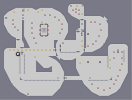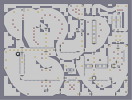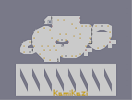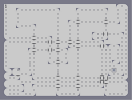weaving weaving extended lights and sounds lights and sounds (editted) Coca-Cola Race Eerie

Pages: (0)

### cuz it was sniped?

get over it, man. i'm not a fan of any band, but i'll still rate 4.5

### this is pretty good

n art but i hate green day....4.5/5

5/5

### kamikaze

i havent forgotten your ded, my level editor is broken...as soon as i fix it you will have a (hopefully) awesome dedication :-)

### I've been waiting for someone to do american idiot

but i thought they would use the tileset somehow. Anyway 5

### BAH

DIE GREEN DAY OMGz but still. its a nice art ill give u that.

### well,

if you want my advice, stay away from bands in general, because some idiot will decide that the band is bad and snipe the map...i like the art (not really the album) but 4.5/5

### ah man i did it again

both of the 'sniper's are supposed to say sniped

### see below

thats meant to say 'sniper'

### omfg

ive just been sniper again

### Awesome

wow thats awesome 5/5 YAH!

4.5/5 too.

4.5/5